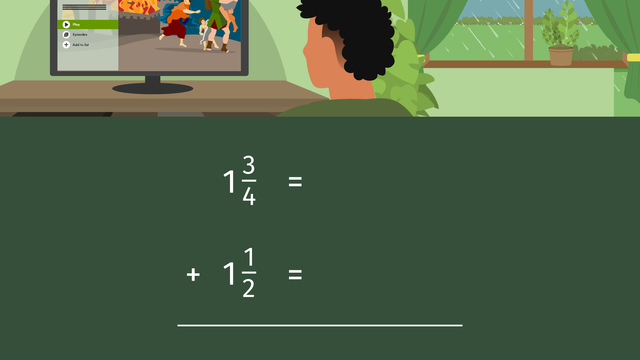# Adding Mixed Numbers with Unlike DenominatorsRating

Be the first to give a rating!
The authorsTeam Digital
Adding Mixed Numbers with Unlike Denominators
CCSS.MATH.CONTENT.5.NF.A.1

## Basics on the topicAdding Mixed Numbers with Unlike Denominators

How do you add mixed numbers with unlike denominators? Find out in this video!

### TranscriptAdding Mixed Numbers with Unlike Denominators

Today is the perfect day! The pouring rain gives us the best excuse to stay indoors. And what's even more exciting? The long-awaited new season of demise has just been released! Time to binge-watch before the spoilers come in and ruin it! We can track how much time we spend watching our favorite show by adding mixed numbers with unlike denominators. Time can be converted into mixed numbers that show fractional amounts that represent parts of a whole, as in hours, or in minutes that represent a portion of an hour. We can add fractional amounts of time to determine the time spent on an activity, like binge watching a series. Let's say it took us one and three-fourths hours to watch episode one, and one and half hours for episode two. We set up the problem like this. To add these mixed numbers, we can to convert them to improper fractions. Starting with this fraction, we multiply the denominator, four by one, and add three to get seven-fourths. In this fraction, we multiply the two by one and add one to get three halves. Now we need to find the least common denominator of four and two, which is four. Seven-fourths would remain the same, and we would multiply three halves by two. The renamed fraction is sixth-fourths. To add these fractions, we bring down the denominator, and add the numerators to get thirteen-fourths. We need to convert this improper fraction back to a mixed number. We do this by dividing the denominator into the numerator. Four divides into thirteen three times, so this becomes our whole number. Four times three is twelve, and thirteen take away twelve is one. One becomes the numerator, and the denominator, four, remains the same. The episodes were watched in three and one-fourth hours. Back to the show for two and three-fourths hours, and after a snack break, one and two-thirds hours more. What is the total number of hours spent on episodes three and four? Let's walk through this one together. You can pause the video at any point if you need extended time. First, convert the mixed numbers to improper fractions. What are the renamed fractions? Eleven-fourths and five-thirds. Now find the least common denominator of four and three. Twelve. What are the renamed fractions with the new numerators? Thirty-three twelfths, twenty-twelfths. What do you get when you add the fractions? Fifty-three twelfths. And now convert it back to a mixed number by dividing the denominator into the numerator. Six and three-twelfths. There is one more step to this answer. Can you see what it is? Three-twelfths can be simplified because three and twelve can be divided by three. What is the final answer for the time spent? Six and one-fourth hours.